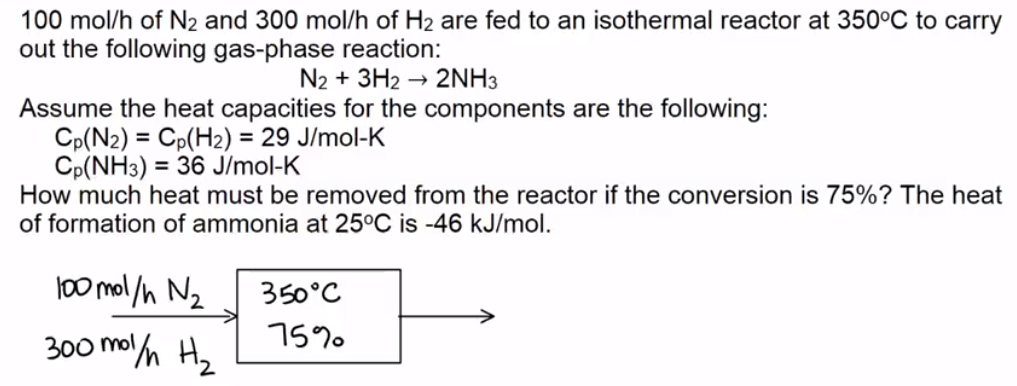LearnChemE

#### Thermal Effects in Chemical Reactions: Example Problems

Try to solve these problems before watching the solutions in the screencasts.

##### Example Problem 1

For the reversible, liquid phase reaction, A↔B, determine the adiabatic equilibrium temperature and the conversion when pure A is fed to the reactor at 300 K.
CPA = CPB = 50 cal/(mol K). Heat capacities are independent of temperature.
The equilibrium constant is 1.0 x
10at 298 K; the heat of the reaction is -2.0 x 104  cal/mol.

##### Example Problem 2

Determine the heat removal so that a chemical reactor is isothermal.